# 指针

1. `int(*match)(void*key1,void*key2);`

1. `match = match_int;`

1. `retval = match(&x,&y);`

# 递归

1. `//facttail.c`
2. `#include"facttail.h"`
3. `int facttail(int n,int a)`
4. `{`
5. `if(n <0)`
6. `return0;`
7. `elseif(0== n)`
8. `return1;`
9. `elseif(1== n)`
10. `return a;`
11. `else`
12. `return facttail( n -1, n * a);`
13. `}`

1. `facttail(n,1);`

# 哈希表

1. `//hashpjw.c`
2. `#include"hashpjw.h"`
3. `//hashpjw`
4. `unsignedint hashpjw(constvoid*key)`
5. `{`
6. `constchar*ptr;`
7. `unsignedint val;`
8. `//hash the key by performing a number of bit operations on it`
9. ` val =0;`
10. ` ptr = key;`
11. `while(*ptr !=‘\0‘)`
12. `{`
13. `unsignedint tmp;`
14. ` val =(val <<4)+(*ptr);`
15. `if( tmp =(val &0xf0000000))`
16. `{`
17. ` val = val ^(tmp >>24);`
18. ` val = val ^ tmp;`
19. `}`
20. ` ptr++;`
21. `}`
22. `//In practice, replace PRIME_TBLSIZ with the actual table size.`
23. `return val % PRIME_TBLSIZ;`
24. `}`

# 表达式树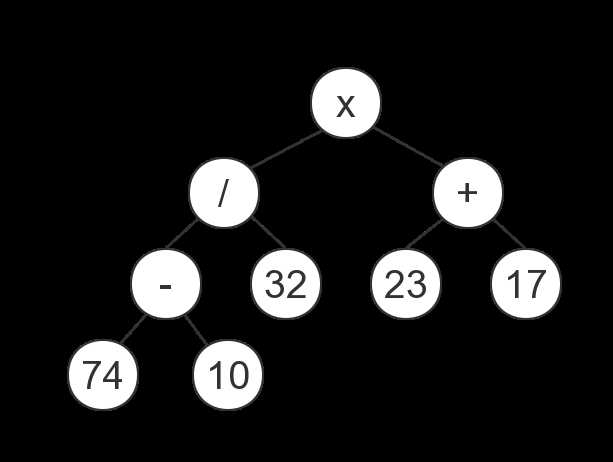(×(/(-74 10)32)(+ 23 17)) = 80

(((74 - 10)/32×(23+17)) = 80

(((74 10 - )32/)(23 17+)× ) = 80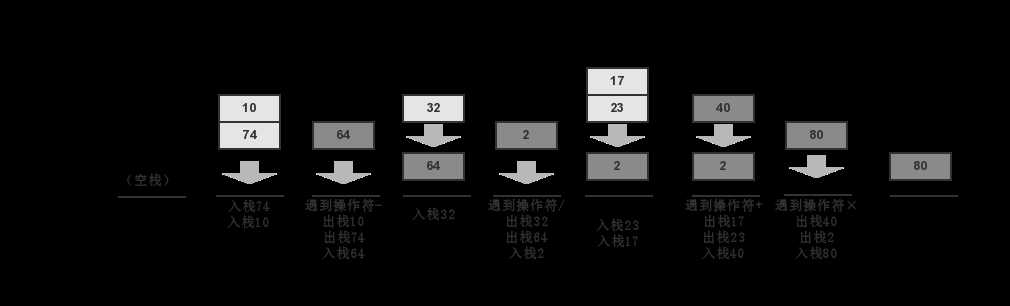# 二叉搜索树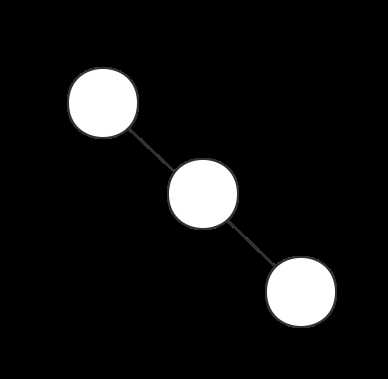# LZ77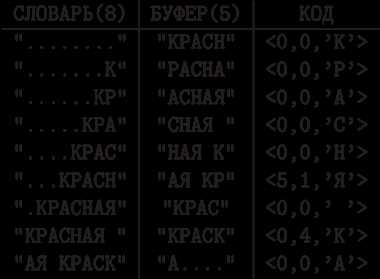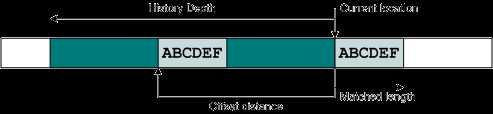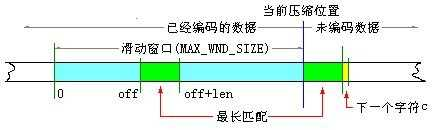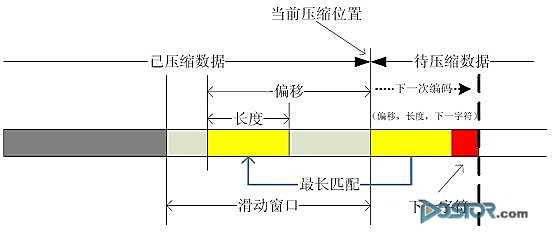12位可以让滑动窗口容纳4KB，在一个32字节的缓冲区中，重复的字节不会超过32个字节，即长度为5位，下一个字节保存在【未匹配的符号】字段内。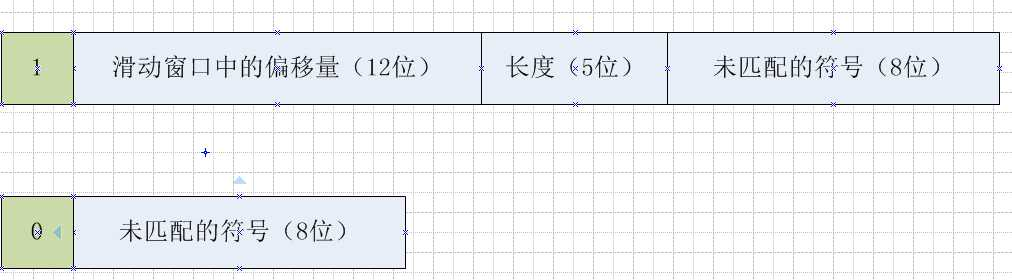# 旅行商问题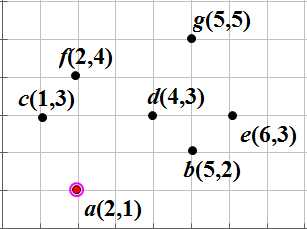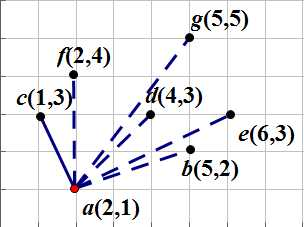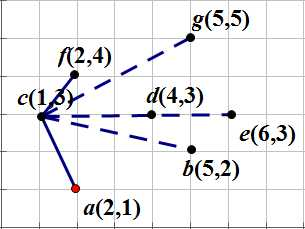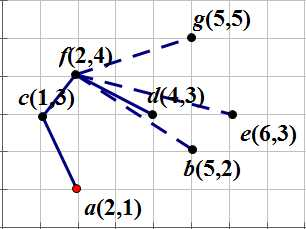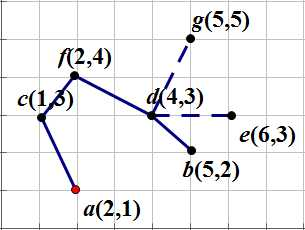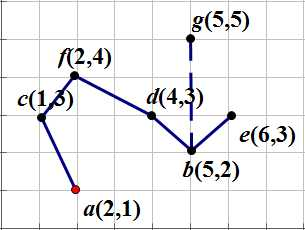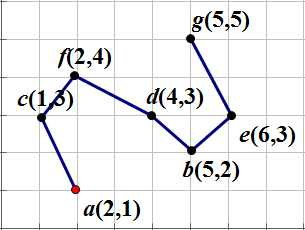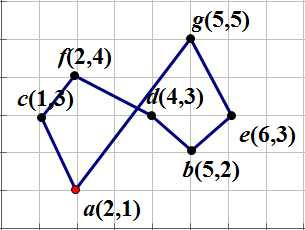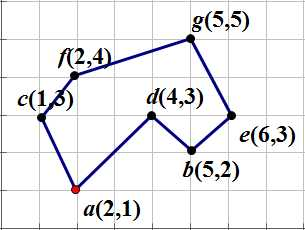# 几何算法

## 凸包

《算法精解 C语言描述》

(0)
(0)

© 2014 bubuko.com 版权所有 鲁ICP备09046678号-4